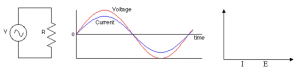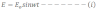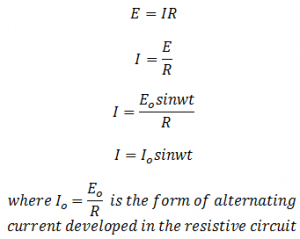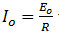# AC Through Resistor OnlyLet an alternating emf be applied to a circuit containing resistor R only such type of circuit is called resistive circuit.
Let the emf applied to the circuit is:Let I be the current in the circuit then potential difference across the resistor is:Comparingwith ohm’s law, we see that current is equal to voltage/resistance
This means the resistance R is resistance for ac which is in fact the resistance for dc. Therefore the behavior of R is same for ac and dc.